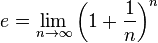# What is this equal to?

$$\lim_{N\rightarrow\infty} \lim_{h\rightarrow 0} \frac{1}{N}\sum_{k=0}^N((kh)^h)^k=$$

and
Nh=L
h = L/N

Hi RandomMystery!$$\lim_ {N\rightarrow +\infty}{\lim_{h\rightarrow 0}{\frac{1}{N}\sum_{k=0}^N{(kh)^{hk}}}}=\lim_ {N\rightarrow +\infty}{\frac{1}{N}\sum_{k=0}^N{\left(\lim_{h \rightarrow 0}{(kh)^h}\right)^k}}$$

So, the first thing you need to do is evaluate

$$\lim_{h\rightarrow 0}{(kh)^h}$$

It seems to go toward 1

Except if k=0, of course. So that leaves us with

$$\lim_{N\rightarrow +\infty}{\frac{1}{N}\sum_{k=1}^N{1}}$$

So, can you evaluate this?

$$\lim_{N\rightarrow +\infty}{\frac{1}{N}\sum_{k=1}^L{1^k}}=1$$

L=Nh (it doesn't let me put two characters on top of the sigma)

It's 1^k right? nvm it always equals 1.
Oh, I think that what I meant was from k=1 or 0 to k=Nh not N on the sigma sign.

So, is what I wrote above right? It can't be right though.

$$\lim_ {N\rightarrow +\infty}{\lim_{h\rightarrow 0}{\frac{1}{N}\sum_{k=0}^N{(kh)^{hk}}}}=\lim_ {N\rightarrow +\infty}{\frac{1}{N}\sum_{k=0}^N{\left(\lim_{h \rightarrow 0}{(kh)^h}\right)^k}}= \frac{\int_0^{L}{x^x}dx}{L}$$

Nh=L

I know that the average value of that function can not be 1 all the time.

I don't know how to prove this though (I just learned series yesterday remember).

Maybe this will fix it...

$$\lim_ {N\rightarrow +\infty}{\lim_{h\rightarrow 0}{\frac{1}{N}\sum_{k=0}^N{(kh)^{hk}}}}=\lim_ {N\rightarrow +\infty}{\frac{1}{N}\sum_{k=0}^N{(\frac{L}{N}k)^{(\frac{Lk}{N})}}}= \frac{\int_0^{L}{x^x}dx}{L}$$

Last edited:
Mute
Homework Helper
Hi RandomMystery!$$\lim_ {N\rightarrow +\infty}{\lim_{h\rightarrow 0}{\frac{1}{N}\sum_{k=0}^N{(kh)^{hk}}}}=\lim_ {N\rightarrow +\infty}{\frac{1}{N}\sum_{k=0}^N{\left(\lim_{h \rightarrow 0}{(kh)^h}\right)^k}}$$

So, the first thing you need to do is evaluate

$$\lim_{h\rightarrow 0}{(kh)^h}$$

The N and h limits are not independent. As stated in the first post, Nh = L = const. The limits cannot be performed separately.

$$\lim_{N\rightarrow +\infty}{\frac{1}{N}\sum_{k=1}^L{1^k}}=1$$

L=Nh (it doesn't let me put two characters on top of the sigma)

It's 1^k right? nvm it always equals 1.
Oh, I think that what I meant was from k=1 or 0 to k=Nh not N on the sigma sign.

So, is what I wrote above right? It can't be right though.

$$\lim_ {N\rightarrow +\infty}{\lim_{h\rightarrow 0}{\frac{1}{N}\sum_{k=0}^N{(kh)^{hk}}}}=\lim_ {N\rightarrow +\infty}{\frac{1}{N}\sum_{k=0}^N{\left(\lim_{h \rightarrow 0}{(kh)^h}\right)^k}}= \frac{\int_0^{L}{x^x}dx}{L}$$

Nh=L

I know that the average value of that function can not be 1 all the time.

I don't know how to prove this though (I just learned series yesterday remember).

Maybe this will fix it...

$$\lim_ {N\rightarrow +\infty}{\lim_{h\rightarrow 0}{\frac{1}{N}\sum_{k=0}^N{(kh)^{hk}}}}=\lim_ {N\rightarrow +\infty}{\frac{1}{N}\sum_{k=0}^N{(\frac{L}{N}k)^{(\frac{Lk}{N})}}}= \frac{\int_0^{L}{x^x}dx}{L}$$

The sum should indeed give you that integral. The integral cannot be performed in terms of elementary functions, so the sum similarly can't be done in closed form.

DArN iT!
I thought I had found a way around it though.
Have you at least tried it this way?

I have another hunch:and look!
$$\lim_ {N\rightarrow +\infty}{\frac{1}{N}\sum_{k=0}^N{(\frac{L}{N}k)^{(\frac{Lk}{N})}}}$$

Which is so close to:

$$\lim_ {N\rightarrow +\infty}{\frac{1}{N}\sum_{k=0}^N{(1+\frac{L}{N}k)^{(\frac{Lk}{N})}}}$$

Maybe we need to define some new function/constant?

$$\lim_ {N\rightarrow \infty}(\frac{L}{N}k)^\frac{Lk}{N}=$$ "f" or "e II"

Last edited:
Mute
Homework Helper
Maybe we need to define some new function/constant?

$$\lim_ {N\rightarrow \infty}(\frac{L}{N}k)^\frac{Lk}{N}=$$ "f" or "e II"

That limit is equivalent to $\lim_{x \rightarrow 0} x^x$, which is 1.

I don't mean to try and stomp on your enthusiasm or creativity, but you should know that there are theorems that show that certain functions, such as x^x, cannot be integrated in terms of elementary functions. See, for example, Liouville's Theorem (differential algebra) and the Risch algorithm. How exactly one shows this I don't know; I haven't really studied these theorems, I am merely aware of their existence.

So, keep playing around with math like this - you likely won't be discovering a closed form integral for x^x in terms of finitely many elementary functions, but maybe you'll discover something else that's cool.